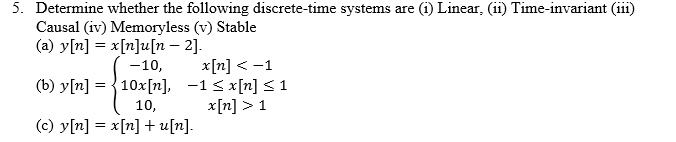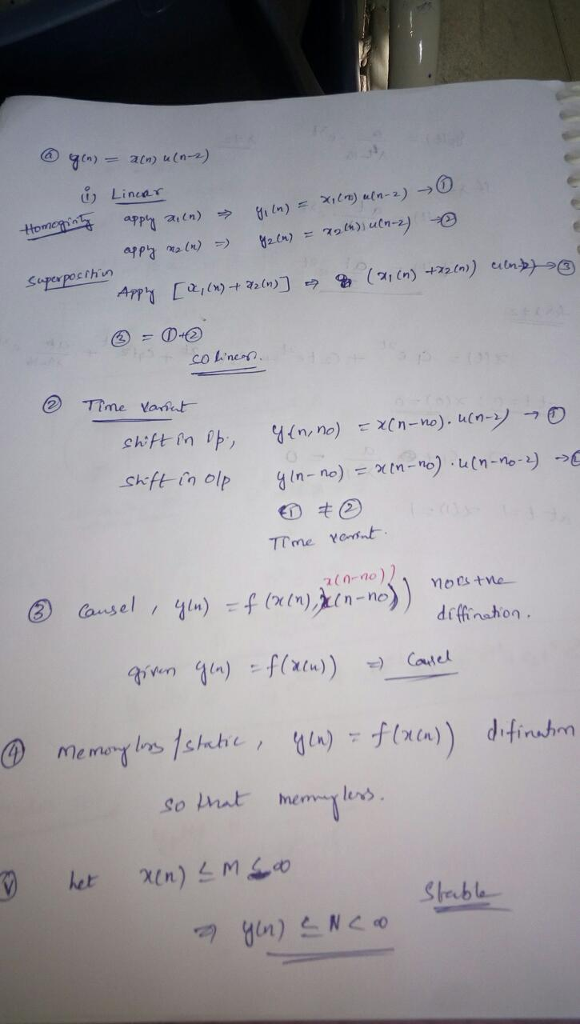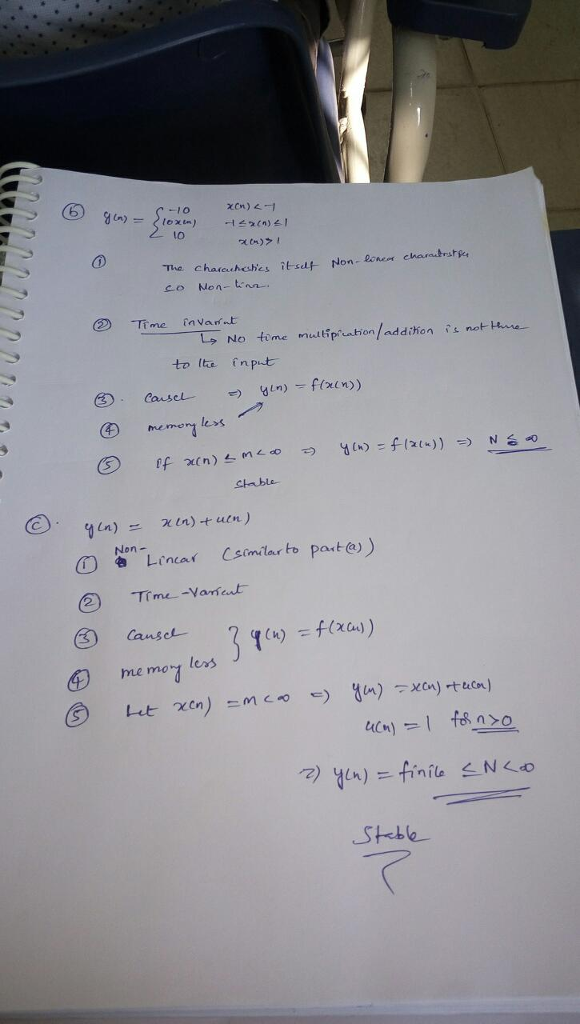# Homework Solution: Determine whether the following discrete-time systems are (i) Linear, (ii) Time-invariant (iii) Causal iv) Mem…Determine whether the following discrete-time systems are (i) Linear, (ii) Time-invariant (iii) Causal iv) Memoryless (v) Stable (a) y[n] = x[n]u[n - 2]. (b) y[n] = { - 10, x[n] 1 (c) y[n] = x[n] + u [n].